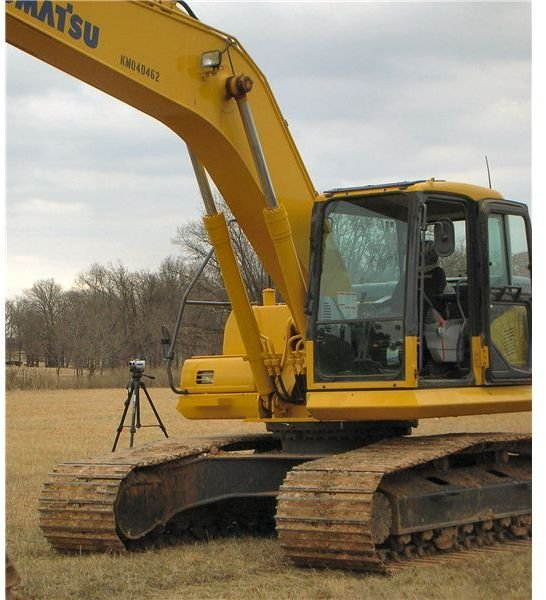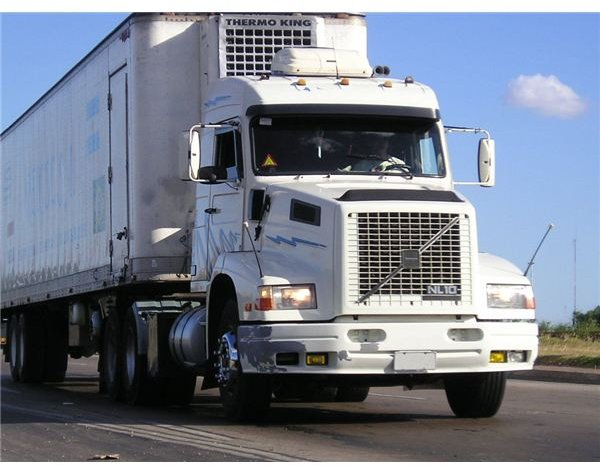An Enterprise Must Calculate Depreciation to Reflect the Use of an Asset Over its Economic Life.

Depreciation of Fixed Assets

Depreciation is an expense charged in the profit and loss account for the consumption of a fixed asset over the period of its useful economic life. The business should calculate depreciation based on the rate at which the economic benefit from the asset is being used up by the business. In this way the consumption of the asset over its useful life is matched with the revenue earned from that asset in the same accounting periods. The estimate of the useful economic life of the asset assumes that it will be regularly maintained and repaired.

In accounting terms depreciation is a non-cash expense that is a debit to the profit and loss account and a credit against the cost of the asset on the balance sheet. Where an asset is revalued on the balance sheet, the depreciation charge applies to the revalued amount of the asset. The balance sheet would normally show the original cost or the market value of the fixed asset, the amount of depreciation charged over its useful life up to the balance sheet date, and the net value of the asset at the balance sheet date.Depreciation is not intended to be a reserve for providing a replacement asset but is intended to show the amount of economic benefit from the asset that has been used up in the accounting period. The depreciation charge therefore represents the expected wear and tear of the asset, or the expected rate at which the asset will become obsolete. This would include technical obsolescence, where more efficient machinery may be developed and used, or commercial obsolescence where the demand for the goods which the asset is used to produce may fall due to changes in fashion or taste. The depreciation charge may also reflect the effect of legal or other restrictions on the use of the fixed asset

The relevant international accounting standard is IAS 16 on Property, Plant and Equipment.The accounting standard asserts that the method of depreciation used should reflect the pattern in which the economic benefits of the asset are used up by the enterprise over its useful life. Under the accounting standard, the residual value and the useful economic life of the asset should be regularly reviewed.

Straight Line Method of Depreciation

A common method of calculating a depreciation charge is to allow for depreciation of the cost of the asset in a straight line over the useful economic life of the asset. The cost or valuation of the asset is therefore divided by the useful economic life and the resulting figure is charged as depreciation in each accounting period. The enterprise may calculate depreciation taking into account an estimated residual value at the end of the asset’s useful life if such a value can be predicted.

Therefore, an item of plant with an estimated useful life of ten years and a cost of \$100,000 dollars would be depreciated at \$100,000 divided by 10 giving an annual straight line depreciation charge of \$10,000 (assuming no residual value). Under this method the enterprise will calculate depreciation assuming that the amount by which the asset depreciates remains constant over its economic life.

Declining Balance (or Reducing Balance) Method

The declining balance methods of depreciation recognise the fact that the depreciation of an asset is unlikely to be constant over its useful life, and that depreciation is likely to be greater during the early years of the useful life of the asset. For example, a business buying a new vehicle will be aware that the market value of that vehicle after it is one year old will have dropped considerably, whereas in subsequent years the drop in value will be less marked.The declining balance method therefore applies a particular percentage to the written down value of the asset to calculate depreciation, that percentage being determined by the estimated useful life of the asset so that its cost or value will have been mostly (but not completely) written down by the end of its useful life. For an asset such as a vehicle that may have an estimated useful life of around ten years, a declining balance method might be applied by writing down the value each year by 25%. Where an asset costs \$40,000, the amount of depreciation in the first year would be 25% of \$40,000 which is \$10,000. This leaves a written down value of \$30,000 for the asset.

In the accounts for the second year of the asset’s useful life, the depreciation would be 25% of \$30,000, being \$7,500. The written down value of the asset on the balance sheet at the end of the second year would therefore be \$22,500. By the tenth year the depreciation charge would be only \$751 and the written down value of the asset at the end of the tenth year would be \$2,252. The declining balance method leaves a residual value at the end of the useful life of the asset, even though this may be very small and may only represent the scrap value of the asset.

Double Declining Balance Method

The double declining balance method of depreciation looks at the percentage depreciation that would be charged under the straight line method of depreciation for a certain asset, and then applies twice that percentage on a declining balance basis. For example, where an enterprise owns an asset with an estimated useful life of ten years it would calculate depreciation at 10% per year under the straight line method, but the double declining balance method would use a 20% declining balance to calculate depreciation.

A variant on the double declining method is the 150% declining balance method which applies the same principle but only takes 150% of the equivalent straight line percentage instead of 200% under the double declining balance method, thereby charging relatively less than the double declining balance method in the early years.

Other Methods of Depreciation

The “sum of the digits” method also recognises that more of the economic benefit of an asset is used up in the earlier years of its life, and therefore charges a greater amount for depreciation in those early years. The method works by taking the estimated useful life of the asset, for example ten years, and then adding up the digits from one to ten to obtain the denominator used to calculate depreciation. The digits from one to ten add up to 55, and in the first year of use of the asset in the trade the depreciation would be computed by dividing ten by 55 and applying the result to the cost or value of the asset. In the second year, nine would be divided by 55 and so on until in the tenth year the depreciation charge would be one fifty-fifth of the cost or value of the asset. Looking at the example of an asset costing \$40,000, the depreciation charge in the tenth year would be only \$727 and the asset would be fully written down at the end of the tenth year of its useful life.

Depreciation might also be allocated among accounting periods during the useful life of an asset based on the expected output of the asset for each period. An enterprise might calculate depreciation, for example, based on estimated production units or operating hours in relation to the asset. In the case of a vehicle, depreciation might be based on the distance travelled in each year of its economic life. Such methods might require more effort to calculate depreciation, but could come closer to allocating the economic benefit of the asset correctly over the accounting periods in which it is used.

Assets on Which No Depreciation is Charged

Certain companies owning fixed assets such as hotels, inns or public houses have considered that they do not need to calculate depreciation on such assets as they are regularly being maintained, repaired and refurbished. However the depreciation charge is a measure of the economic benefit of the asset that has been used in the year. The only situation in which depreciation would not need to be included would be if the amount of depreciation on a particular asset or group of assets did not amount to a material figure in the accounts. This might be the case where the expected useful economic life of the asset was very long, for example more than fifty years. In all other cases depreciation should be charged.